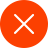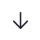-www.mx816.com-

# 周华健

## 产品详情

----------------------------------------------------------------------------------------------------------------------------------------------------------------------------------------------------------------------------------------------------------------------------------------

--------------------------------------------------------------------------------------------------------------------------------------------

▪ 生活 ( 1996.10.20)     ▪ 今夜阳光灿烂 ( 1994.7)     ▪ 有故事的人 ( 1998.8.5)
▪ 世界由你我开始 ( 1997.10.20)     ▪ 爱的光 ( 1996.2.2)     ▪ 爱相随 ( 1995.7.7)
▪ 风雨无阻 ( 1994.6.15)     ▪ 弦弦全全 ( 1995.12.22)     ▪ 弦犹在耳 ( 1996)
▪ 光阴似健 ( 1997)     ▪ 现在 ( 1999.11.1)     ▪ 小天堂 ( 1996.7.12)
▪ 弦途有你 ( 1995.5.9)     ▪ 有弦相聚 ( 1994.4.27)     ▪ 忘忧草 ( 2001.10.16)
▪ 心的方向 ( 1987.7.13)     ▪ 让我欢喜让我忧 ( 1991.12.3)     ▪ 一起吃苦的幸福 ( 2003.3.30)
▪ 周而复始 ( 2003.10)     ▪ 雨人 ( 2006.3.25)     ▪ 至爱吾爱 ( 1999.2)
▪ 不愿一个人 ( 1990.12.19)     ▪ 我愿意去等 ( 1995.1.21)     ▪ 最真的梦 ( 1988.11.1)
▪ 期待更多付出更多 ( 1989.3.16)     ▪ 花心 ( 1993.4.30)     ▪ NOW现在 ( 1999.11.30)
▪ 花旦 ( 2011.5.20)     ▪ 难说再见 ( 2010.11.1)     ▪ 雨人 ( 2006.3.25)
▪ 男人三十 ( 2005.9.10)     ▪ 一起吃苦的幸福 ( 2003.3.30)     ▪ NOW现在 ( 1999.11.30)
▪ 小天堂 ( 1996.1.1)     ▪ 爱相随 ( 1995.7.7)     ▪ 我愿意去等 ( 1995.1.21)
▪ 花心 ( 1993.4.1)     ▪ 期待更多付出更多 ( 1989.3.16)     ▪ 最真的梦 ( 1988.11.1)

▪ 心的方向 ( 1987.7.13)长按图片保存/分享13757718326（微信同步）327807726@qq.comCopyright @ VillaGrandis All Rights Reserved 浙ICP备14034553号-1

• 取消